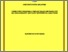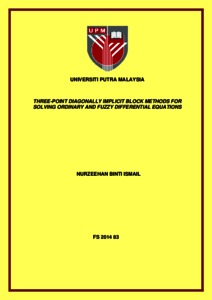# Three-point diagonally implicit block methods for solving ordinary and fuzzy differential equations

## Citation

Ismail, Nurzeehan (2014) Three-point diagonally implicit block methods for solving ordinary and fuzzy differential equations. Masters thesis, Universiti Putra Malaysia.

## Abstract

The focus of this thesis is on the derivations of Diagonally Implicit Block Backward Differentiation Formulas (DBBDF) of constant step size. The first part of the thesis discusses on the modification of Fully Implicit Block Backward Differentiation Formulas (FBBDF) to solve first order fuzzy differential equations (FDEs). The subsequent part of the thesis focuses on the derivations of Diagonally Implicit Three-point BBDF of order two and three (DBBDF (3, 2) and DBBDF (3, 3)) for solving first order ordinary differential equations (ODEs) and FDEs. The convergence properties for DBBDF methods and the adequate stability regions for the proposed methods are presented to show that the methods are capable of solving stiff ODEs. The derived methods are then implemented using Newton iteration which is normally used since the methods derived are implicit in nature. Numerical results are presented to verify the efficiency of DBBDF methods for ODEs. The derived methods are then compared with Diagonally Implicit Two-point BBDF of order two, three and four (DBBDF (2, 2), DBBDF (2, 3) and DBBDF (2, 4)). The accuracy of the proposed methods outperformed DBBDF (2, 3) and DBBDF (2, 4) as the step size gets smaller while the computational time of the proposed methods are smaller than the existing methods. Since there are very few block methods used to solve fuzzy differential equations, the derived methods are extended to solve first order fuzzy initial value problems (FIVPs). The fuzzification of DBBDF methods is proposed and the convergence of the corresponding methods when applied to FDEs is also proven. Numerical results of DBBDF methods when solving FDEs are provided and compared with the existing methods. On the whole, this study reveals that the FBBDF and DBBDF methods are capable and efficient for solving first order ordinary and fuzzy differential equations.Preview
Text
FS 2014 83 - IR.pdfView Item